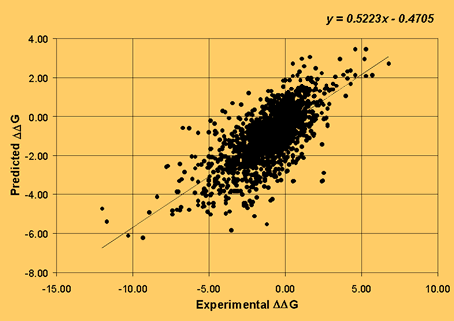I-Mutant2.0
 I-Mutant2.0 Help Last Update 27/12/06

I-Mutant2.0: a tool for predicting protein stability upon mutation

Introduction

I-Mutant2.0 is a Support Vector Machine -based web server for the automatic prediction of protein stability changes upon single-site mutations. The tool was trained on a data set derived from ProTherm  that is presently the most comprehensive database of experimental data on protein mutations. Our predictor can evaluate the stability change upon single site mutation starting from the protein structure or from the protein sequence. When trained/tested with a cross validation procedure, I-Mutant2.0 correctly predicts whether the protein mutation stabilises or destabilises the protein in 80% of the cases when the three-dimensional structure is known and 77% of the cases when only the protein sequence is available.

Results

In the table some scoring indexes of the efficiency of the method are listed. The protein stability change is predicted from the structure (I-Mutant2.0-PDB) or from the sequence (I-Mutant2.0-Seq).

 Q2 P(+) Q(+) P(-) Q(-) C I-Mutant2.0-PDB 0.80 0.73 0.56 0.83 0.91 0.51 I-Mutant2.0-Seq 0.77 0.69 0.46 0.79 0.91 0.42

The overall accuracy Q2 is:

Q2=p/N

where p is the total number of correctly predicted residues and N is the total number of residues.
The correlation coefficient C is defined as:

C(s)=[ p(s)n(s)-u(s)o(s) )] / D

where D is the normalization factor

D =[(p(s)+u(s))(p(s)+o(s))(n(s)+u(s))(n(s)+o(s))]1/2

for each class s (+ and -, for increasing and decreasing stability, respectively); p(s) and n(s) are the total number of correct predictions and correctly rejected assignments, respectively, and u(s) and o(s) are the numbers of under and over predictions.

The coverage for each discriminated structure s is evaluated as:

Q(s)=p(s)/[ p(s)+u(s)]

where p(s) and u(s) are as defined above. The probability of correct predictions P(s) (or accuracy for s) is computed as:

P(s)=p(s) / [p(s) + o(s)]

where p(s) and o(s) are previously defined (ranging from 1 to 0).

I-Mutant2.0 predicts also the value of the stability change upon single point mutation. In this particular task it reaches a correlation with the experimental data of 0.71 (see figure below) when structural information is considered (Standard Error is 1.3 Kcal/mol) and 0.62 when only the protein sequence is available (Standard Error is 1.45 Kcal/mol).Required Inputs

I-Mutant2.0 is optimized to predict the protein stability change upon mutation either starting from the protein structure (Task 1) or the protein sequence (Task 2). In both cases, the end-user can predict the protein stability change corresponding to all possible mutations of a particular residue, or ask only for a specific mutation. In either case, I-Mutant2.0 can predict the direction of the free energy change and its value. If the end-user requires only one single prediction, instead of 19 possible mutations in a given position, the option “New residue” may be selected.

Task 1) When the structure of the protein under study is known the following inputs are necessary:

• PDB code: the PDB protein code ;
• Chain: if the input is a PDB file containing more than one chain, the chain label is also necessary; otherwise the default value is "_";
• Position: the PDB position number of the residue that undergoes mutation;
• Temperature: the Temperature value in Celsius degrees [0-100];
• pH: the negative logarithm value of H+ concentration [0-14].
Task 2) When only the protein sequence is available the required inputs are:

• Protein Sequence: the protein sequence in raw format and one letter code;
• Position: the position number in the sequence of the residue that undergoes mutation;
• Temperature: the Temperature value in Celsius degrees [0-100];
• pH: the negative logarithm value of H+ concentration [0-14].

For either prediction the option is to predict the sign (increase +, decrease -) of the free energy change (DDG) or its value (+/- DDG) upon mutation. The results can be sent to your e-mail address, if you ask for it, or obtained interactively if you do not past your e-mail in the proper box. For more details see the tutorial web pages.

Outputs

The output consists of a table listing the sign or the value of the predicted stability changes upon the 19 possible mutations for a given PDB or sequence position. This allows you to select the most appropriate mutation for the position at hand. If the box “New Residue” has been activated, only the results corresponding to a given mutation will be returned.

The RSA value (Relative Solvent Accessible Area) can be calculated with the DSSP program  only when prediction is structure-based, dividing the accessible surface area value of the mutated residue by the free residue surface .

The RI value (Reliability Index) is computed only when the sign of the stability change is predicted and is evaluated from the output of the support vector machine O as

RI=20*abs(O-0.5).

The DDG value is calculated from the unfolding Gibbs free energy value of the mutated protein minus the unfolding Gibbs free energy value of the wild type (Kcal/mol).

WARNING:
Possible errors may occur when the PDB files contain broken chains or the numbering for the selected residue is different from than expected by the user. Numbering is crucial also when stability changes are predicted from the protein sequence.
Sometimes the prediction of the sign of DDG can be discordant to the prediction of DDG value. It is due to the fact that the predictions are performed with two different SVM, anyway this occours only when the Reliability Index (RI) of the prediction is low.

 Bava KA, Gromiha MM, Uedaira H, Kitajima K, Sarai A. (2004). ProTherm, version 4.0: thermodynamic database for proteins and mutants. Nucleic Acids Res. 32, D120-D121.

 Berman HM, Westbrook J, Feng Z, Gilliland G, Bhat TN, Weissig H, Shindyalov IN, Bourne PE (2000). The Protein Data Bank. Nucleic Acids Res. 28, 235-242.

 Kabsch W, Sander C (1983). Dictionary of protein secondary structure: pattern of hydrogen-bonded and geometrical features. Biopolymers. 22, 2577-2637.

 Chothia C (1976). The Nature of the Accessible and Buried Surfaces in Proteins. J. Mol. Biol. 105, 1-14.